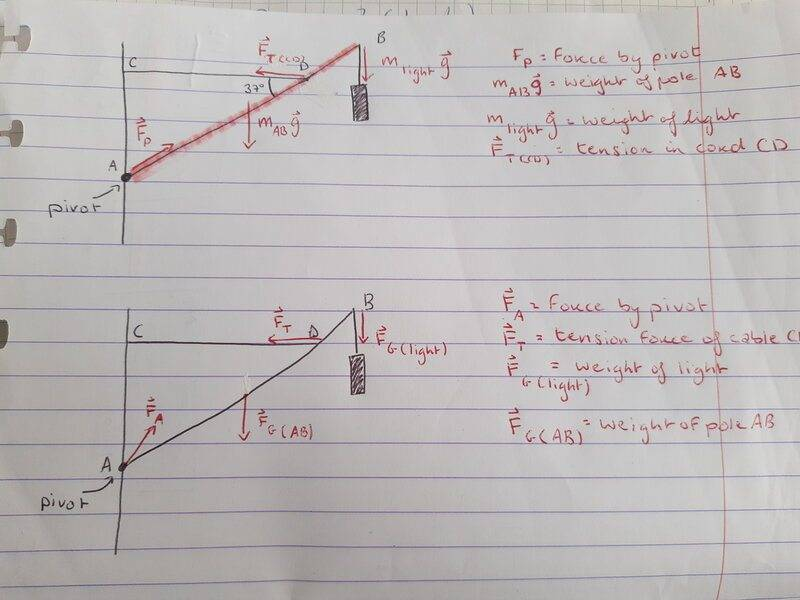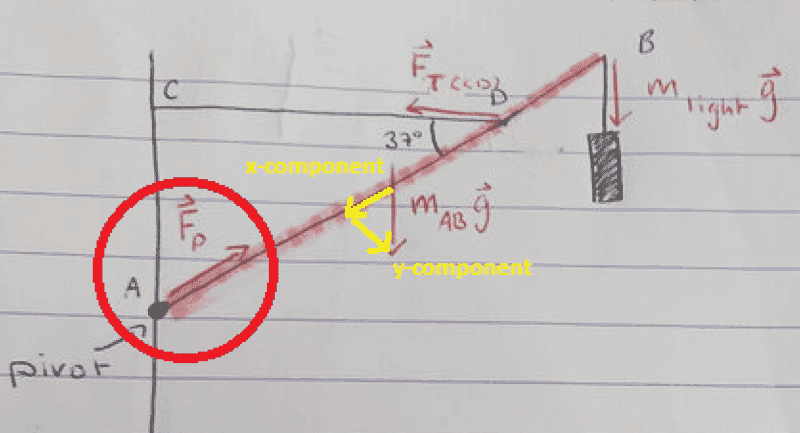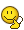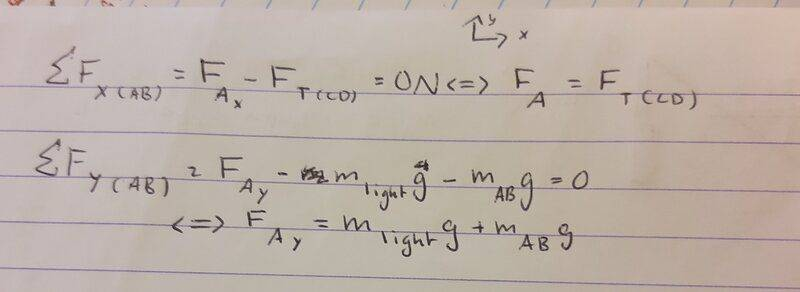# Is the force exerted by a pivot always towards the center of mass?

• lela
Ah, do you mean that I need to use the fact that the net torque must be zero to calculate the tension in the cable?f

#### lela

Homework Statement
A traffic light hangs from a pole as shown in Fig. 12–60. The uniform aluminum pole AB is 7.20 m long and has a mass of 12.0 kg. The mass of the traffic light is 21.5 kg.
Determine (a) the tension in the horizontal massless cable CD, and (b) the vertical and horizontal components of the force exerted by the pivot A on the aluminum pole.
Relevant Equations
Newton's second law for translational and rotational motion, also think Newton's third law might be relevant.
I thought that the force by the pivot A on the pole AB would be the reaction force to the x-component of the gravitational force on AB. This would mean that the force by the pivot would be parallel to the pole, but in my notes from class the force vector seems to be more along the bisector of CÂB..

I checked in my textbook and found an example question about the force by a floor on a ladder, here I assumed that the pivot would be at the point where the ladder touches the floor and again thought that the force by the floor would be parallel to the ladder, but in the figure it showed the force as being closer to the bisector and said something about the force not having to act along the direction of the ladder because the ladder is rigid, which doesn't make it more clear to me.. (link to figure https://ibb.co/DwLJrMW)

I looked at another question in my textbook with this figure (link https://ibb.co/vsr80h5) where they ask for the force by the hinge on the beam and in the figure for the answer it shows the force by the hinge not being along the beam (like I would have assumed, link https://ibb.co/kMZk30K).

So now I'm trying to figure out if my initial reasoning is correct (that the force by the pivot is a reaction force to the weight), and if not, how I can understand where the froce by the pivot originates from/how to identify it.

Welcome! :)

Which pole do you refer to when you write "This would mean that the force by the pivot would be parallel to the pole, but in my notes from class the force vector seems to be more along the bisector of CÂB."

Have you created a free body diagram of the componets of this armature that could show us?

Welcome! :)

Which pole do you refer to when you write "This would mean that the force by the pivot would be parallel to the pole, but in my notes from class the force vector seems to be more along the bisector of CÂB."

Have you created a free body diagram of the componets of this armature that could show us?
Thank you for the welcome and the reply!

I highlighted the pole that I was talking about there in pink.
Here are the free body diagrams, the top one is the one that I thought would be right and the bottom one is the one from my class notes (different vectors for the force from A/pivot), I drew the forces acting on the pole (pink) AB.•Lnewqban
So now I'm trying to figure out if my initial reasoning is correct (that the force by the pivot is a reaction force to the weight), and if not, how I can understand where the froce by the pivot originates from/how to identify it.
The thing a pivot cannot do is resist any torque.

The thing a pivot cannot do is resist any torque.
Sorry, I don't understand. Am I supposed to take torques in consideration when looking for the force exerted by a pivot onto a pole?

Sorry, I don't understand. Am I supposed to take torques in consideration when looking for the force exerted by a pivot onto a pole?
Yes, taking the moment (torque) about the pivot gives you an important equation. If the pivot was a solid arm welded to the post, then you wouldn't need the cable BC.

Yes, taking the moment (torque) about the pivot gives you an important equation. If the pivot was a solid arm welded to the post, then you wouldn't need the cable BC.
But I thought there was no torque about a pivot since the distance from the exerted force to the pivot is zero

But I thought there was no torque about a pivot since the distance from the exerted force to the pivot is zero
You must be misunderstanding something. There must be zero torque about that pivot point. That's one of your force balancing equations.

Also, because the pivot cannot resist a torque, you need the cable BC to keep the traffic light from crashing to the ground. If there were no pivot and the whole sructure were rigid, you wouldn't need the cable.

You must be misunderstanding something. There must be zero torque about that pivot point. That's one of your force balancing equations.

Also, because the pivot cannot resist a torque, you need the cable BC to keep the traffic light from crashing to the ground. If there were no pivot and the whole sructure were rigid, you wouldn't need the cable.
Ah, do you mean that I need to use the fact that the net torque must be zero to calculate the tension in the cable? If so, I understood this part. I am confused about how to calculate the force exerted by the pivot onto the pole AB (part b of the question) and why it is not parallel to the pole AB as I have drawn/assumed in this diagram. Is it not a reaction force to the x-component of the weight of AB that is directed at the pivot?I am confused about how to calculate the force exerted by the pivot onto the pole AB (part b of the question) and why it is not parallel to the pole AB as I have drawn/assumed in this diagram.
The reaction force at the pivot could be in any direction. Imagine taking hold of that arm AB and pushing or pulling it however you like. The pivot must react to that by Newton's third law. That force is an unknown in your equations.

•lela
The reaction force at the pivot could be in any direction. Imagine taking hold of that arm AB and pushing or pulling it however you like. The pivot must react to that by Newton's third law. That force is an unknown in your equations.
Aaaah, that makes sense. I see now how it was wrong to think of it as only a reaction to the weight of AB, dang it seems so obvious nowSo since the structure here is stable I can assume the net forces on AB in the x and y directions are zero, and then use this together with all the other forces acting on AB to find the the x- and y-components of the force by the pivot? Like this:Thank you a lot again! And sorry for taking so long to get what you were saying•Lnewqban